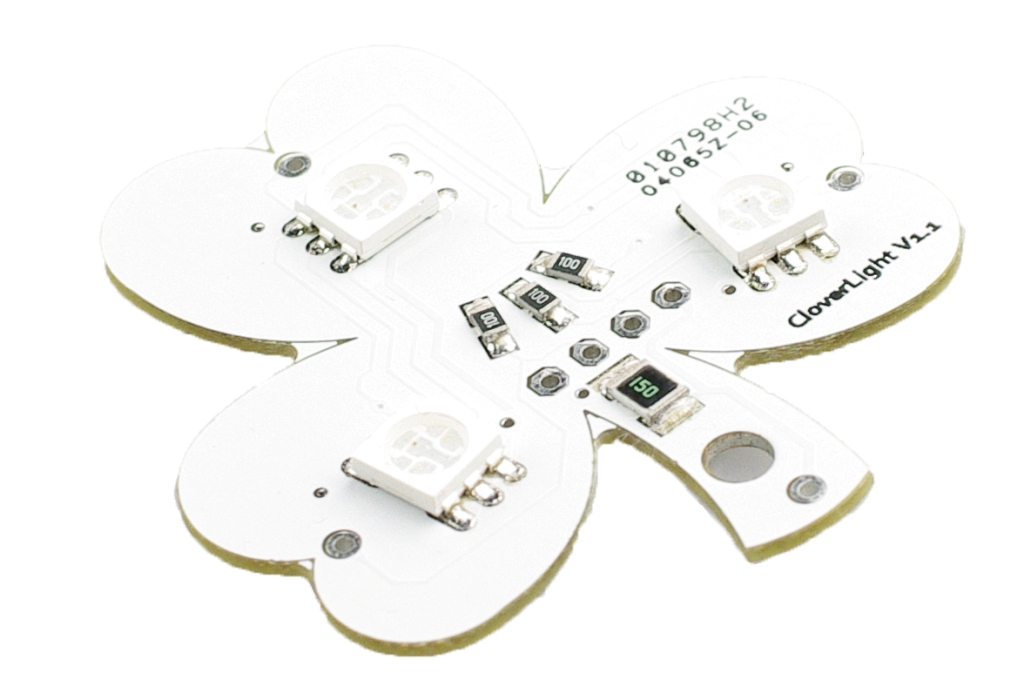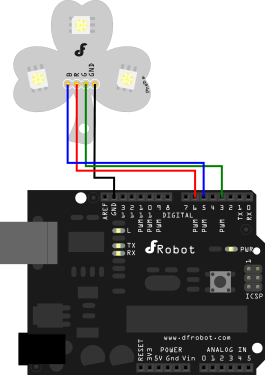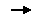## Introduction

The clover light is a new RGB LED board from DFRobot. This board connects to PWM pins for control. By pulse-width-modulating the pins any color can be created by mixing different amounts of red, green, and blue. Running from a 5V source, these LEDs are very bright.

Applications are unlimited: LED Video Screen array, Signage, PC Case Illumination, Bar/Club lighting, Festive Lighting, Garden Lighting, Arduino project application, and decorations.

### Specification

Power supply: 5v Built in resistors Arduino compatible Size: 42x40x5mm

### Shipping List

Clover Light (1 unit) Right Angle pin header 4p (1 unit)

## Connection Diagram## Sample Code

``````// #
// # Editor     : Phoebe from DFRobot
// # Date       : 2012.3.21

// # Product name: Clover Light v1.2
// # Product SKU : DFR0190
// # Version     : 1.0

// # Description:
// # The colorful sketch for the rgb led

// # Connection:
// #        LED driver pins -> D3,D5,D6(PWM pins)
// #        GND -> Arduino GND
// #

byte LightDiscPins;

void setup()
{

byte LightPins = {      //R G B using the pwm pins
6,3,5       };
InitLightDiscPins(LightPins);

//  Serial.begin(57600);

}

void loop()
{

for(int j = 0;j < 360; j++){
DiscLightUpHSV(j,255,255);//*******
delay(30);
}

}

void InitLightDiscPins(byte pins[]){

for(int i = 0; i < 3; i ++)  LightDiscPins[i] = pins[i];
analogWrite(LightDiscPins,255);
analogWrite(LightDiscPins,255);
analogWrite(LightDiscPins,255);

}

void DiscLightUp(int red, int green, int blue){

analogWrite(LightDiscPins,constrain(red,0,255));
analogWrite(LightDiscPins,constrain(green,0,255));
analogWrite(LightDiscPins,constrain(blue,0,255));
}

void DiscLightUpHSV(int hue, int val, int sat){

int __max_brightness = 255;
uint8_t sector = hue / 60;
uint8_t rel_pos = hue - (sector * 60);
uint16_t const mmd = 255 * 255;     /* maximum modulation depth */
uint16_t top;
top = val * 255;
uint16_t bottom = val * (255 - sat);    /* (val*255) - (val*255)*(sat/255) */
uint16_t slope = (uint16_t)(val) * (uint16_t)(sat) / 120;   /* dy/dx = (top-bottom)/(2*60) -- val*sat: modulation_depth dy */
uint16_t a = bottom + slope * rel_pos;
uint16_t b = bottom + (uint16_t)(val) * (uint16_t)(sat) / 2 + slope * rel_pos;
uint16_t c = top - slope * rel_pos;
uint16_t d = top - (uint16_t)(val) * (uint16_t)(sat) / 2 - slope * rel_pos;
uint16_t R, G, B;

if (sector == 0)    {
R = c;
G = a;
B = bottom;
}
else if (sector == 1)   {
R = d;
G = b;
B = bottom;
}
else if (sector == 2)   {
R = bottom;
G = c;
B = a;
}
else if (sector == 3)   {
R = bottom;
G = d;
B = b;
}
else if (sector == 4)   {
R = a;
G = bottom;
B = c;
}
else    {
R = b;
G = bottom;
B = d;
}

uint16_t scale_factor = mmd / __max_brightness;

R = (uint8_t) (R / scale_factor);
G = (uint8_t) (G / scale_factor);
B = (uint8_t) (B / scale_factor);

DiscLightUp(R,G,B);
}``````Go Shopping [Clover Light (SKU:DFR0190)]